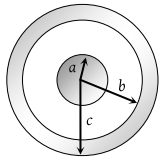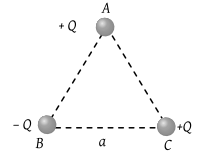Two charges each of 1 coulomb are at a distance 1 km apart, the force between them is

(1) 9 × 103 Newton

(2) 9 × 10–3 Newton

(3) 1.1 × 10–4 Newton

(4) 104 Newton

Concept Questions :-

Coulomb's law
High Yielding Test Series + Question Bank - NEET 2020

Difficulty Level:

+2C and +6C two charges are repelling each other with a force of 12 N. If each charge is given –2C of charge, then the value of the force will be

(1) 4 N (Attractive)

(2) 4 N (Repulsive)

(3) 8 N (Repulsive)

(4) Zero

Concept Questions :-

Coulomb's law
High Yielding Test Series + Question Bank - NEET 2020

Difficulty Level:

The dielectric constant of pure water is 81. Its permittivity will be

(1) 7.17 × 10–10 MKS units

(2) 8.86 × 10–12 MKS units

(3) 1.02 × 1013 MKS units

(4) Cannot be calculated

Concept Questions :-

Coulomb's law
High Yielding Test Series + Question Bank - NEET 2020

Difficulty Level:

Force of attraction between two point charges Q and – Q separated by d meter is Fe. When these charges are placed on two identical spheres of radius R = 0.3 d whose centres are d meter apart, the force of attraction between them is

(1) Greater than Fe

(2) Equal to Fe

(3) Less than Fe

(4) Less than Fe

Concept Questions :-

Coulomb's law
High Yielding Test Series + Question Bank - NEET 2020

Difficulty Level:

One metallic sphere A is given positive charge whereas another identical metallic sphere B of exactly same mass as of is given equal amount of negative charge. Then

(1) Mass of A and mass of B is the same

(2) Mass of A is more

(3) Mass of B is less

(4) Mass of B is more

Concept Questions :-

Ways of charging
High Yielding Test Series + Question Bank - NEET 2020

Difficulty Level:

The force between two charges 0.06 m apart is 5 N. If each charge is moved towards the other by 0.01 m, then the force between them will become

(1) 7.20 N

(2) 11.25 N

(3) 22.50 N

(4) 45.00 N

Concept Questions :-

Coulomb's law
High Yielding Test Series + Question Bank - NEET 2020

Difficulty Level:

When 1019 electrons are removed from a neutral metal plate, the electric charge on it is

(1) –1.6 C

(2) +1.6 C

(3) 10+19 C

(4) 10–19 C

Concept Questions :-

Ways of charging
High Yielding Test Series + Question Bank - NEET 2020

Difficulty Level:

Two charges each equal to 2μC are 0.5m apart. If both of them exist inside vacuum, then the force between them is

(1) 1.89 N

(2) 2.44 N

(3) 0.144 N

(4) 3.144 N

Concept Questions :-

Coulomb's law
High Yielding Test Series + Question Bank - NEET 2020

Difficulty Level:

A solid conducting sphere of radius a has a net positive charge 2Q. A conducting spherical shell of inner radius b and outer radius c is concentric with the solid sphere and has a net charge –Q. The surface charge density on the inner and outer surfaces of the spherical shell will be(1) $-\frac{2Q}{4\pi {b}^{2}},\frac{Q}{4\pi {c}^{2}}$

(2) $-\frac{Q}{4\pi {b}^{2}},\frac{Q}{4\pi {c}^{2}}$

(3) $0,\frac{Q}{4\pi {c}^{2}}$

(4) None of the above

Concept Questions :-

Gauss law
High Yielding Test Series + Question Bank - NEET 2020

Difficulty Level:

Three charges are placed at the vertices of an equilateral triangle of side ‘a’ as shown in the following figure. The force experienced by the charge placed at the vertex A in a direction normal to BC is(1) ${Q}^{2}/\left(4\pi {\epsilon }_{0}{a}^{2}\right)$

(2) $-{Q}^{2}/\left(4\pi {\epsilon }_{0}{a}^{2}\right)$

(3) Zero

(4) ${Q}^{2}/\left(2\pi {\epsilon }_{0}{a}^{2}\right)$

Concept Questions :-

Coulomb's law